Choose Language Hide Translation Bar
Revised in JMP 10: Power Analysis in Custom Design

In my previous post, I talked about the fundamental quantities that affect the ability of a designed experiment to detect non-negligible effects of the factors. These are:

1)      The size of the effect

2)      The root mean squared error (RMSE) of the fitted model

3)      The significance level of the hypothesis test

4)      The number of runs

Here, I introduce the new Power Analysis user interface in JMP 10.

Why did you make a change?

It is always a bit risky to change the interface of an existing feature of software, so we did it with some trepidation. However, as you will see, the new interface offers more capability and clarity (we hope).

So what’s different?

Figure 1 shows the JMP 9 and JMP 10 interfaces side by side. The design being evaluated is an orthogonal two-level design with six factors and 12 runs.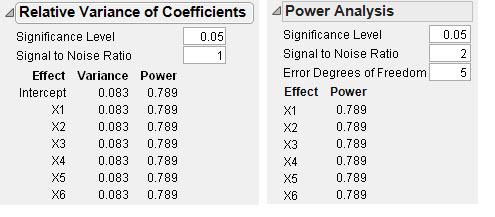The first thing you might notice is that the name of the outline node has changed from Relative Variance of Coefficients to Power Analysis. Since many practitioners must demonstrate that a proposed design has adequate power before proceeding, having Power Analysis easily visible seemed like a good idea.

Note that the reported power for all the factors is the same in both versions but the Signal to Noise Ratio is 1 in JMP 9 and 2 in JMP 10.

What’s up with that?

In JMP 10, we have re-defined what we mean by the signal in Signal to Noise Ratio. In JMP 9, the signal was defined as the magnitude of regression coefficient in Fit Model. Now, for two-level factors, we define the signal as the magnitude of the difference in the predicted response going from one level to the other. In other words, the signal is the effect of the factor. The factor effect is twice the regression coefficient for two-level factors. To make the reported power be the same, we needed to double the default Signal to Noise Ratio. So the size of the default effect has not changed, only the definition of the Signal to Noise Ratio.

There is an extra edit box for the Error Degrees of Freedom in JMP 10. By default, this value is the difference between the number of runs and the number of parameters. There is one exception to this rule and that is when the number of runs is equal to the number of parameters. In that case, there are actually no error degrees of freedom, and technically you cannot test the effect of any factor so the power would be undefined. In this case, we “invent” one degree of freedom, so that we can provide some meaningful (albeit generally depressingly low) value for power.

What is the utility of the Error Degrees of Freedom edit box? Isn’t changing that number cheating?

It is not cheating unless you increase the number of error degrees of freedom without subsequently increasing the number of runs in the design to match!

There are two uses for allowing control of the Error Degrees of Freedom.

First, by increasing the error degrees of freedom, you can get a quick and dirty assessment of how the power will increase if you add runs to your design. And you can find this out without generating a new design.

Second, you can use the error degrees of freedom to get a more accurate picture of the power for detecting the effect of a whole plot factor in a split-plot experiment. This is something that required JSL scripting in JMP 9.

How does that work?

Consider a scenario with two factors that are hard to change from one run to another. Suppose you have six factors in all. You decide to do 16 runs in groups of two where the hard to change factors stay the same in each group. Figure 2 shows the Custom Design setup.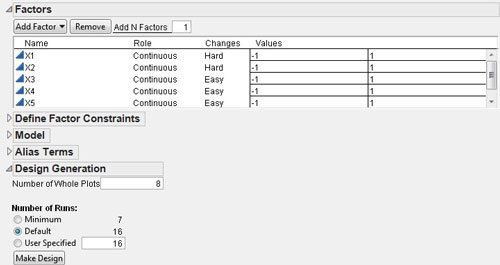If you click the Make Design button, you get an orthogonal split-plot design. Before looking at the Power Analysis section, I recommend going to the red triangle menu and choosing Simulate Responses. Then click the Make Table button. Finally, run the Model script in the top left panel of the resulting table.

Figure 3 shows the Parameter Estimates from the fake data I generated. The parameter estimates are not important. I want to draw your attention to the column of the table labeled DF Den.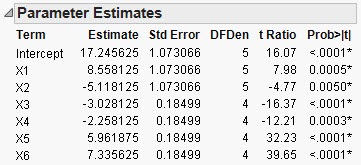X1 and X2 are the Whole Plot factors. They have 5 degrees of freedom for error. X3 through X6 are the subplot factors, and they each have 4 degrees of freedom for error.

Recall that the default Error Degrees of Freedom is the difference between the number of runs and the number of parameters. In this case, that number is 9. Figure 4 shows the default Power Analysis interface.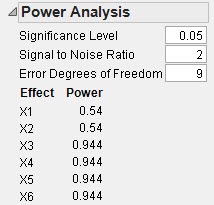To get the correct values for the power of the whole plot effects, we should change the Error Degrees of Freedom to 5. Similarly, for the subplot effects, we should change the Error Degrees of Freedom to 4. Figure 5 shows the side-by-side result.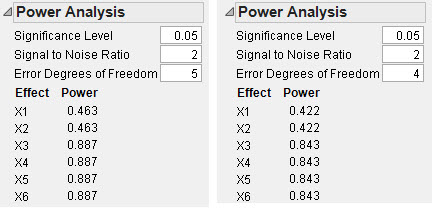So, the power for detecting whole plot effects in this design is 0.463 – see the top two numbers on the left panel of Figure 5. The power for detecting subplot effects is 0.843 – see the bottom four numbers on the right panel of Figure 5. This analysis assumes that the whole plot variance and the error variance are the same (a standard assumption).

Why couldn’t JMP do all this?

Unless the design is orthogonal, the degrees of freedom shown in the REML analysis depend on the observed responses. So, in general, it is not easy to fill in these values in advance. Stay tuned for further improvements in JMP 11.

Are there other differences?

The Power Analysis has a number of hidden columns. Figure 6 shows the complete table for the first example. You add these columns to the display by right-clicking inside the table.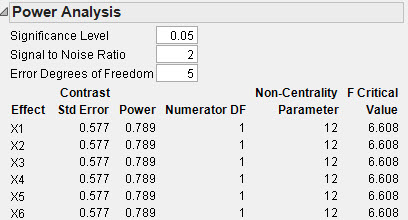The extra columns provide information that would allow you to do these calculations by hand using a JSL script.

//Power = 1 - F Distribution( F Crit, dfnum, dfdenom, nonCentrality );

Power = 1 - F Distribution( 6.608, 1, 5, 12 );

Running the above JSL script outputs 0.789140997559235 to the Log window.

What’s next?

So far I have only talked about factors with two levels. With more than two levels, the situation is more complicated. That will be the subject of a future post.

Article Labels

There are no labels assigned to this post.

Visitor

Jake Warren wrote:

So, for clarification, are these two interpretations correct?

In JMP 9: Power is the probability of finding a significant model parameter if the model parameter is X times as large as the error standard deviation, where X is the specified Signal to Noise Ratio. In other words, power is the probability of finding a significant model parameter if the change in the mean response across levels of a factor is 2X times larger than the error standard deviation.

In JMP 10: Power is the probability of finding a significant model parameter if the model parameter is .5X times as large as the error standard deviation, where X is the specified Signal to Noise Ratio. In other words, power is the probability of finding a significant model parameter if the change in the mean response across levels of a factor is X times larger than the error standard deviation.

Visitor

Jake Warren wrote:

I'm not sure I said what I wanted to above. I think this words the statements better.

In JMP 9: Power is the probability of finding a significant model parameter if the change in the mean response across levels of a factor (the effect size) is 2*X*Error Standard Deviation, or the regression coefficient is X*Error Standard Deviation, where X is the Signal to Noise Ratio.

In JMP 10: Power is the probability of finding a significant model parameter if the change in the mean response across levels of a factor (the effect size) is X*Error Standard Deviation, or the regression coefficient is .5*X*Error Standard Deviation, where X is the Signal to Noise Ratio. (Essentially, here, the Signal to Noise Ratio is double what it was in JMP 9.)

Visitor

Ryan wrote: